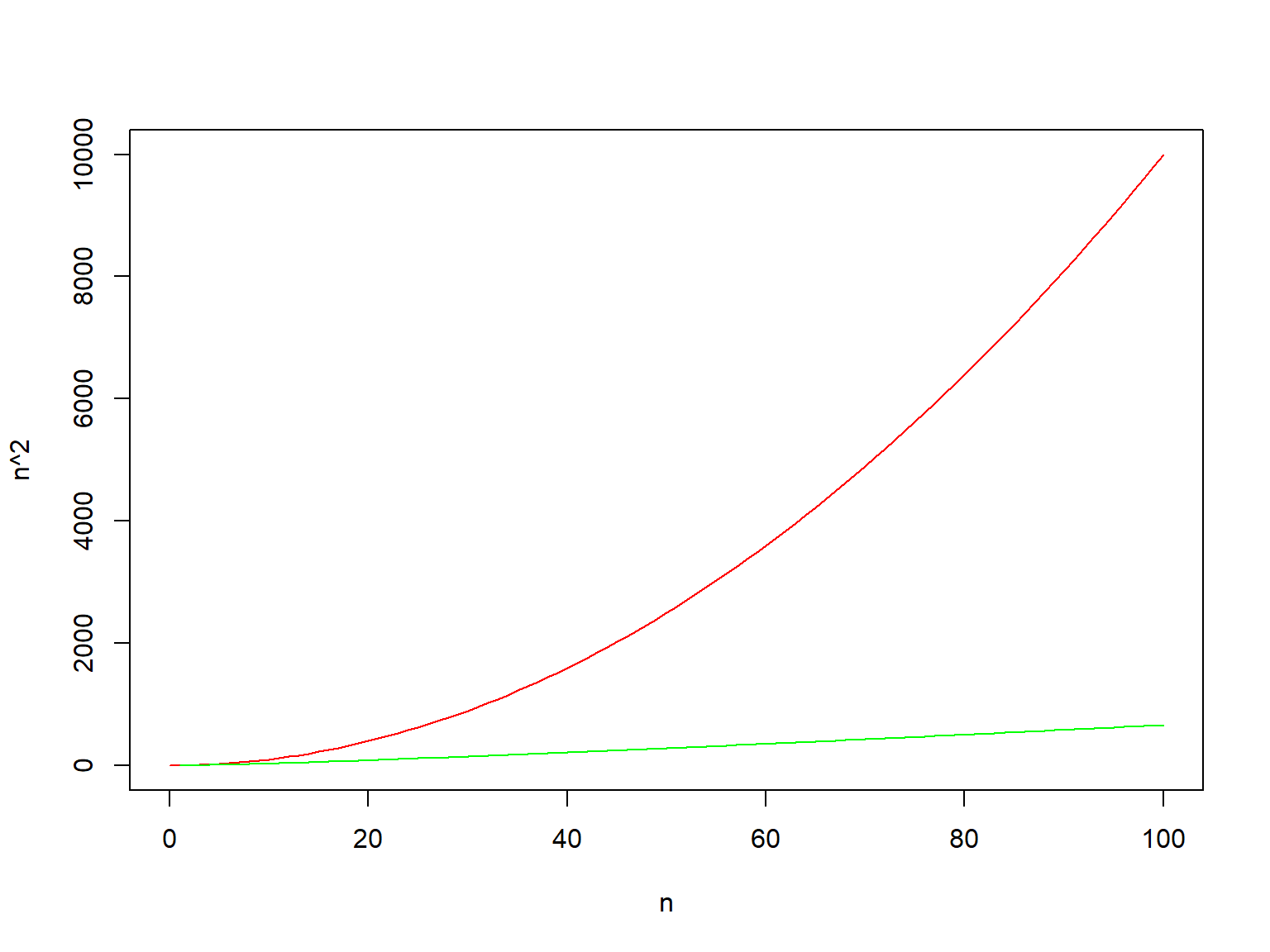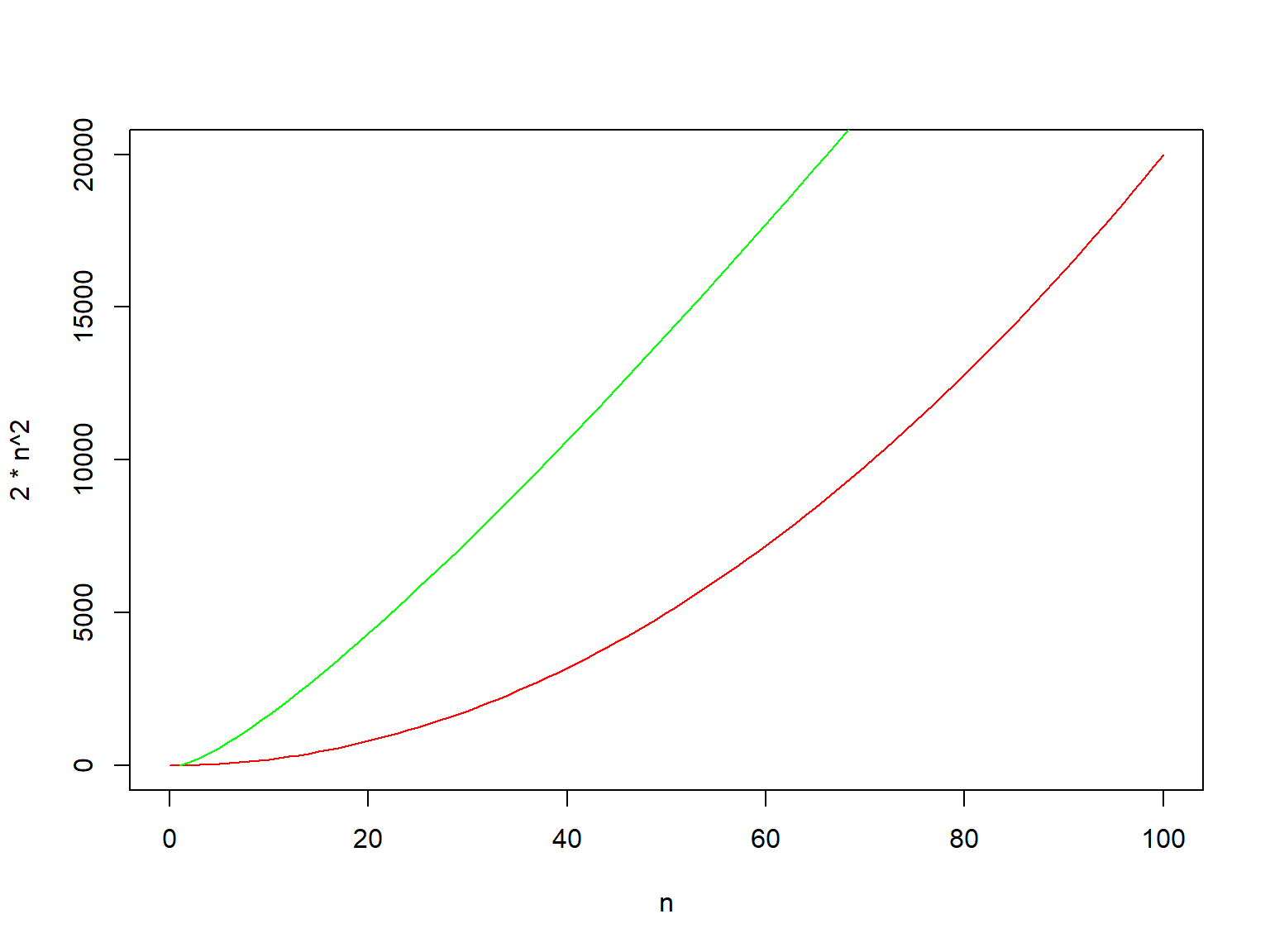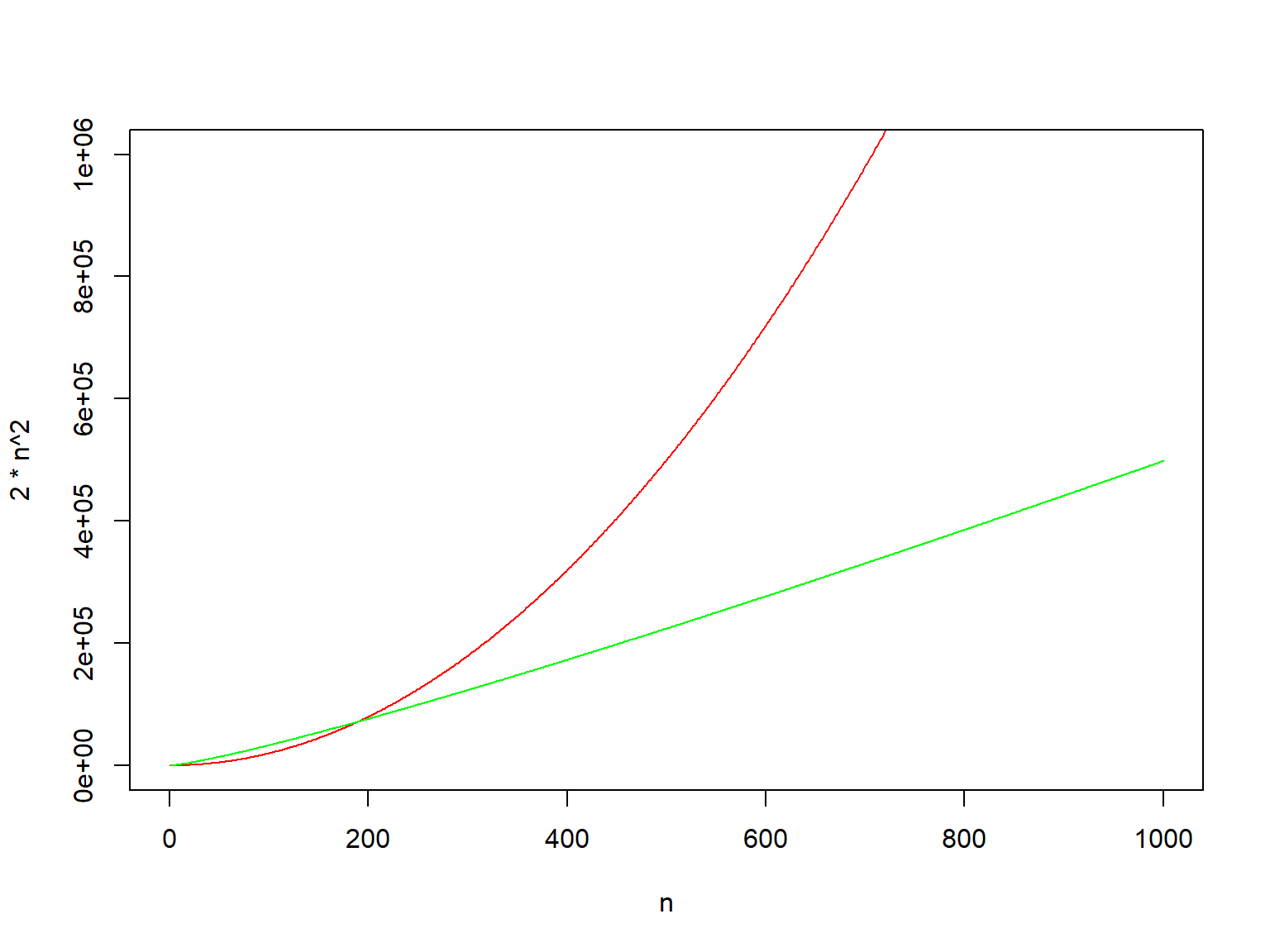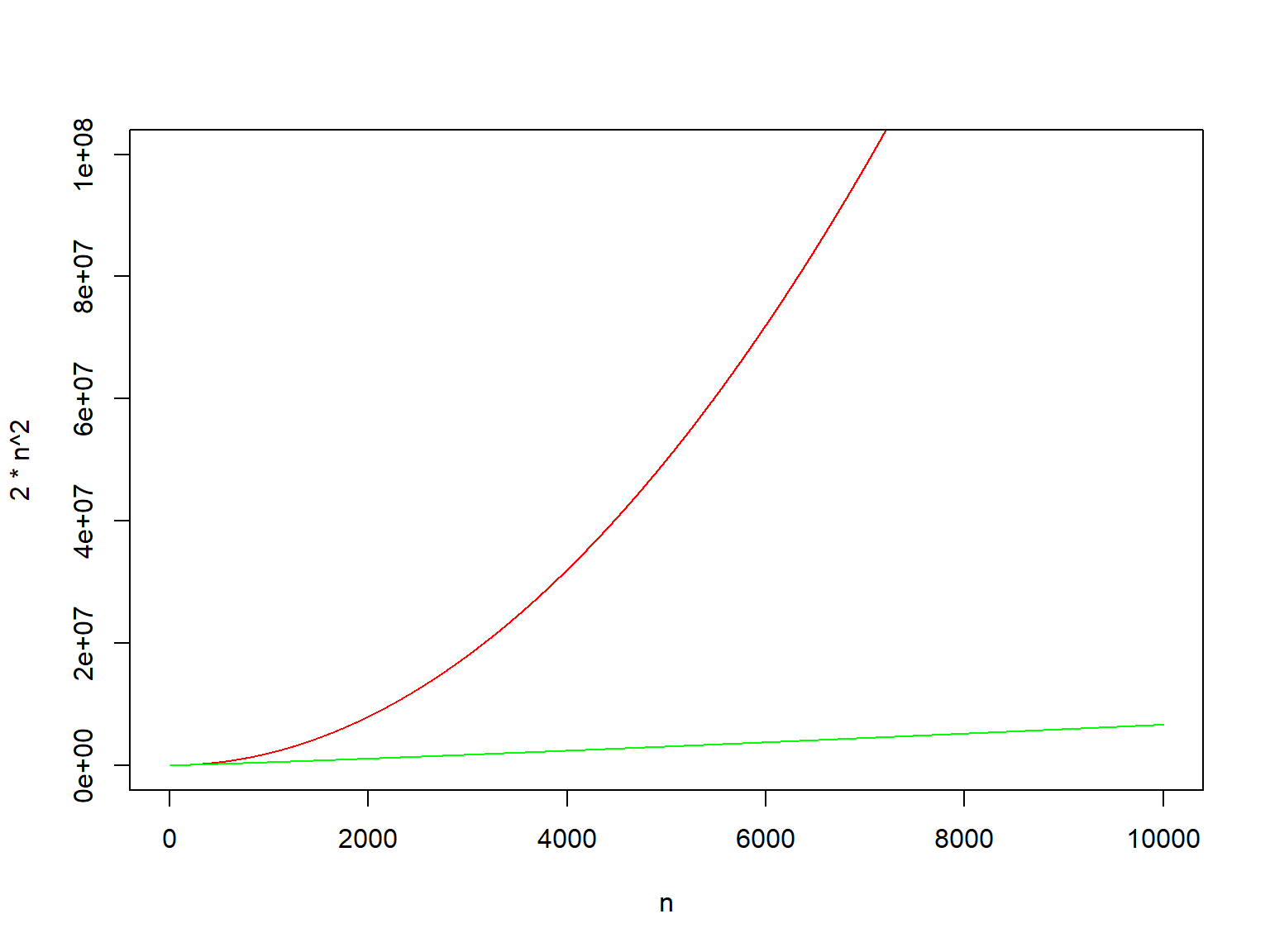July 17, 2019

# Algorithms

• These slides are based on the book:
• Introduction to Algorithms
• Thomas H. Cormen, Charles E. Leiserson, Ronald L. Rivest, and Clifford Stein
• The MIT Press, Third Edition
• ISBN: 978-0-262-03384-8 (hardcover: alk. paper)
• ISBN: 978-0-262-53305-8 (pbk: alk. paper)

# Algorithms

• Algorithm
• Informally: any well defined computational procedure
• Input values
• Output values
• A computational sequence of steps that transform an input into an output
• Algorithm describes such a transformation
• Tool to solve a well specified computational problem

# Algorithms

• Sorting problem
• Input: sequence of numbers $$(a_1, a_2, \dots, a_n)$$
• Output: permutation (reordering), $$(a'_1, a'_2, \dots, a'_n)$$ of the input sequence such that $$a'_1 \leq a'_2 \leq \dots \leq a'_n$$.
• For sequence (31, 41, 59, 26, 41, 58), the output is:
• (26, 31, 41, 41, 58, 59)

# Algorithms

• The input sequence is known as instance
• An instance
• Satisfies the problem restrictions
• Necessary to compute the problem solution

# Algorithms

• Sorting is a fundamental operation in computer science
• Used as an intermediate step
• There are many good sorting algorithms
• Which one is the best for a given task?
• It depends…
• Number of elements to sort?
• Are some of the elements already sorted?
• Are there restrictions in the values to sort?
• What kind of storing are we using (main memory, hard drives, tapes)?
• We’ll work with different sorting algorithms

# Algorithms

• Correct, an algorithm is correct $$iff$$ for each input instance, it halts when it obtains the correct output
• We say that a correct algorithm solves the given computational problem
• An incorrect algorithm
• Might not halt at all for some instances
• Might halt with an incorrect answer
• Incorrect algorithms might be usefull
• If we can control their error rate
• We’ll work with correct algorithms

# Algorithms

• Algorithm specification
• Can be specified in English
• As a computer program
• As pseudocode
• There’s only one restriction
• Specification must provide a precise description of the comptational procedure to follow

# Algorithms

• There’s a lot more than sorting algorithms
• The Human Genome project
• Identify 100,000 genes from human DNA
• The sequence has 3 billion of chemical base pairs that make up human DNA
• Internet search engines, large volumen of data
• Electronic comerce, electronic transactions, security (cryptography)
• Manufacturing and other commercial enterprises that need to allocate scarce resources in the most beneficial way

# Algorithms

• Common characteristics of algorithms
• They have many candidate solutions but most of them are useless for us, finding the one we need might be difficult
• They have practical applications, as in the shortest path

# Algorithms

• Data structures
• A way to store and organize data to facilitate access and modification
• A data structure doesn’t work well for every purpose, they have strenghts and limitations

# Algorithms

• Technique
• It might be that the algorithm we need isn’t published yet
• We need a technique to design and analyze algorithms
• So we can develop new ones
• Show they produce the right answer
• Understand their efficiency

# Algorithms

• Hard Problems
• There exist some problems for which an efficient solution isn’t known
• NP-complete algorithms
• No efficient algorithm has been found for NP-complete problems
• We don’t know either whether there exist or not efficient algorithms for NP-complete problems

# Algorithms

• Property of NP-complete algorithms
• If there exists an efficient algorithm for any of them, then there exist efficient algorithms for all of them
• Several NP-complete problems are similar (not identical) to problems for which we know there exist efficient algorithms
• A subtle change in the problem definition causes a big change in the efficiency of the best known algorithm

# Algorithms

• It’s good to know about NP-complete problems
• They appear frequently in real world applications
• We may invest too much time in them
• If we show that the algorithm is NP-complete
• We could invest all that time in developing an algorithm that gets a good answer (not the best possible one)

# Algorithms

• Parallelism
• For many years single processor clock speeds increased at steady rate
• But there are physical limitations on ever increasing clock speeds
• Chips have been designed to to contain more than one core
• Multicore computers are a type of parallel computer

# Algorithms as a Technology

• What would happen if computer were infinitely fast and memory was free?
• Oh!, but since they aren’t infinitely fast nor memory is free
• Computing time and memory space are limited resources
• We must be smart to use them
• Need efficient algorithms in time and space

# Algorithms as a Technology

• Efficiency
• Algorithms solving the same problem frequently have different efficiency
• More significant than differences due to HW or SW

# Algorithms as a Technology

• Efficiency, sorting algorithms
• InsertionSort $$c_1n^2$$ to sort $$n$$ elements, $$c_1$$ is a constant independent from $$n$$
• MergeSort $$c_2n \lg$$$$n$$, where $$\lg$$$$n$$ is $$\log_2$$$$n$$ and $$c_2$$ is another constant independent of $$n$$
• $$c_1 < c_2$$
• constant factors are not as important as $$n$$
• $$n$$ much higher than $$\lg$$$$n$$
• InsertionSort faster than MergeSort for small inputs
• There is a point (in size of $$n$$) in which MergeSort gets faster than InsertionSort

# Algorithms as a Technology

• Efficiency, example
• Computer A is faster than Computer B
• A, 100 millions of instructions per second $$\rightarrow 10^8$$ inst/sec
• B, 1 million of instructions per second $$\rightarrow 10^6$$ inst/sec
• We implement InsertionSort in A, $$2n^2$$ ($$c_1 = 2$$)
• We implement MergeSort in B, $$50n$$$$\lg$$$$n$$ ($$c_2 = 50$$)
• Task of sorting 1,000,000 of numbers, ($$n = 10^6$$)

# Algorithms as a Technology

• $$A \rightarrow \frac{2(10^6)^2 inst}{10^8 inst/sec}$$ = 20,000 seconds = 5.56 hours
• $$B \rightarrow \frac{50 \cdot 10^6 \lg 10^6 inst}{10^6 inst/sec}$$ = 1,000 seconds = 16.67 minutes
• With 10,000,000 numbers
• B (20 min) is 20 times faster than A (2.3 days) to sort the numbers
• The advantage of the efficiency of MergeSort

# Algorithms as a Technology

• We can plot the functions to see how they behave with different sizes of the input, n
• $$y_1 = n^2$$ (in red in the plot)
• $$y_2 = n lg n$$ (in green in the plot)
• This plot shows values for $$n = 1 \dots 100$$
n<-seq(0,100,1)
plot(n,n^2, type="l", col="red")
lines(n,n*log2(n), col="green")# Algorithms as a Technology

• Ah!, we need to consider the constants for both functions
• $$y_1 = 2n^2$$ (in red in the plot)
• $$y_2 = 50n lg n$$ (in green in the plot)
• This plot shows values for $$n = 1 \dots 100$$
n<-seq(0,100,1)
plot(n,2*n^2, type="l", col="red")
lines(n,50*n*log2(n), col="green")# Algorithms as a Technology

• What happens with larger input sizes?
• $$y_1 = 2n^2$$ (in red in the plot)
• $$y_2 = 50n lg n$$ (in green in the plot)
• This plot shows values for $$n = 1 \dots 1000$$
n<-seq(0,1000,1)
plot(n,2*n^2, type="l", col="red", xlim=range(0,1000), ylim=range(0,1000000))
lines(n,50*n*log2(n), col="green")# Algorithms as a Technology

• What happens with even larger input sizes?
• $$y_1 = 2n^2$$ (in red in the plot)
• $$y_2 = 50n lg n$$ (in green in the plot)
• This plot shows values for $$n = 1 \dots 10000$$
n<-seq(0,10000,1)
plot(n,2*n^2, type="l", col="red", xlim=range(0,10000), ylim=range(0,100000000))
lines(n,50*n*log2(n), col="green")# Algorithms as a Technology

• Algorithms and other technologies
• Predict the resources required by the algorithm
• Memory, computing time
• Consider the model of the implementation technology
• 1 processor
• RAM memorry
• One instruction after the other, no concurrent operations
• We need mathematical tools to describe/represent this!